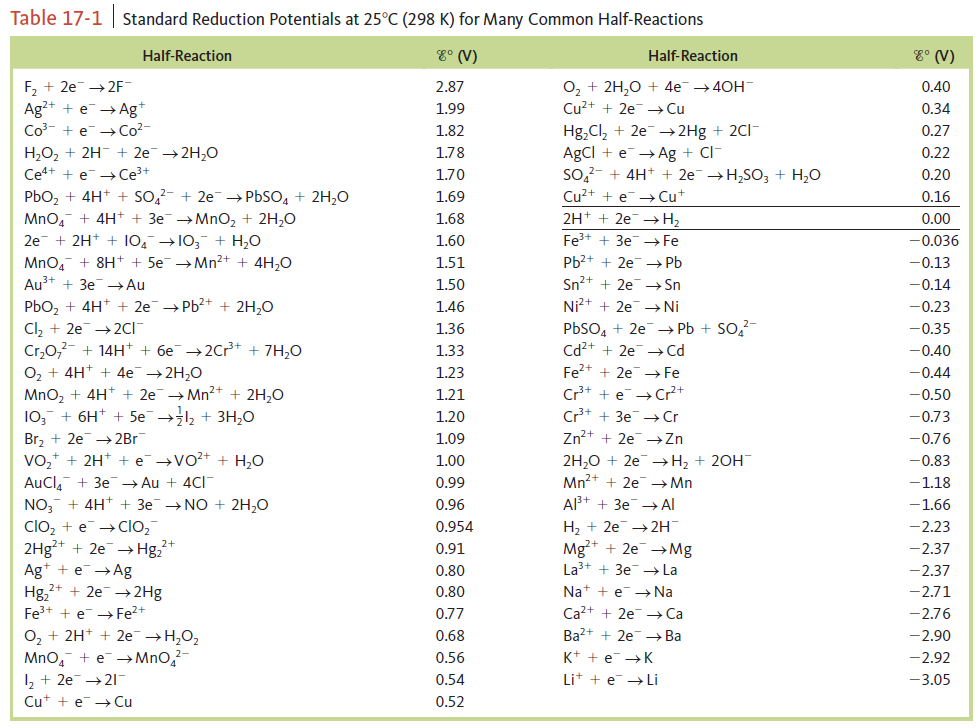# Problem: Chlorine dioxide (ClO2), which is produced by the reaction 2NaClO2 (aq) + Cl2 (g) → 2ClO2 (g) + 2NaCl (aq)has been tested as a disinfectant for municipal water treatment. Using data from Table 17‑1, calculate E° and ΔG° at 25°C for the production of ClO2.

###### FREE Expert Solution

Calculate E°cell.

Recall:

Lose               Gain
Electron         Electrons
Oxidation       Reduction

cathode → reduction → oxidation number decreases

anode → oxidation → oxidation number increases

For ions: charge of the ion = oxidation number
For neutral atoms/compound: oxidation number = 0

We have:

ClO2- ClO(oxidation number decreases)

Cl→ 2 Cl- (oxidation number decreases)

From the reduction potential table, we have:

ClO+ e- → ClO2-                             E°cell = 0.954 V                           anode

Cl+ 2 e- → 2 Cl-                              E°cell = 1.36 V.                            cathode

$\overline{){\mathbf{E}}{{\mathbf{°}}}_{{\mathbf{cell}}}{\mathbf{=}}{\mathbf{E}}{{\mathbf{°}}}_{{\mathbf{cathode}}}{\mathbf{-}}{\mathbf{E}}{{\mathbf{°}}}_{{\mathbf{anode}}}}\phantom{\rule{0ex}{0ex}}\mathbf{E}{\mathbf{°}}_{\mathbf{cell}}\mathbf{=}\mathbf{1}\mathbf{.}\mathbf{36}\mathbf{V}\mathbf{-}\mathbf{0}\mathbf{.}\mathbf{954}\mathbf{V}$

91% (1 ratings)###### Problem Details

Chlorine dioxide (ClO2), which is produced by the reaction

2NaClO2 (aq) + Cl2 (g) → 2ClO2 (g) + 2NaCl (aq)

has been tested as a disinfectant for municipal water treatment. Using data from Table 17‑1, calculate E° and ΔG° at 25°C for the production of ClO2.Frequently Asked Questions

What scientific concept do you need to know in order to solve this problem?

Our tutors have indicated that to solve this problem you will need to apply the Cell Potential concept. If you need more Cell Potential practice, you can also practice Cell Potential practice problems.

What professor is this problem relevant for?

Based on our data, we think this problem is relevant for Professor Massa's class at HUNTER.

What textbook is this problem found in?

Our data indicates that this problem or a close variation was asked in Chemistry: An Atoms First Approach - Zumdahl Atoms 1st 2nd Edition. You can also practice Chemistry: An Atoms First Approach - Zumdahl Atoms 1st 2nd Edition practice problems.